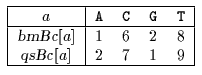Next: Raita algorithm Up: ESMAJ Prev: Berry-Ravindran algorithm

# Smith algorithm

Main features
• takes the maximum of the Horspool bad-character shift function and the Quick Search bad-character shift function;
• preprocessing phase in O(m+) time and O() space complexity;
• searching phase in O(mn) time complexity.
Description

Smith noticed that computing the shift with the text character just next the rightmost text character of the window gives sometimes shorter shift than using the rightmost text character of the window.
He advised then to take the maximum between the two values.

The preprocessing phase of the Smith algorithm consists in computing the bad-character shift function (see chapter Boyer-Moore algorithm) and the Quick Search bad-character shift function (see chapter Quick Search algorithm).

The preprocessing phase is in O(m+) time and O() space complexity.

The searching phase of the Smith algorithm has a quadratic worst case time complexity.

The C code

The function preBmBc is given chapter Boyer-Moore algorithm and the function preQsBc is given chapter Quick Search algorithm.

```void SMITH(char *x, int m, char *y, int n) {
int j, bmBc[ASIZE], qsBc[ASIZE];

/* Preprocessing */
preBmBc(x, m, bmBc);
preQsBc(x, m, qsBc);

/* Searching */
j = 0;
while (j<= n - m) {
if (memcmp(x, y + j, m) == 0)
OUTPUT(j);
j += MAX(bmBc[y[j + m - 1]], qsBc[y[j + m]]);
}
}

```
The example

Preprocessing phasebmBc and qsBc tables used by Smith algorithm.

Searching phase

References
• SMITH P.D., 1991, Experiments with a very fast substring search algorithm, Software - Practice & Experience 21(10):1065-1074.Next: Raita algorithm Up: ESMAJ Prev: Berry-Ravindran algorithm

e-mails: {Christian.Charras, Thierry.Lecroq }@laposte.net
Tue Jan 14 15:03:31 MET 1997